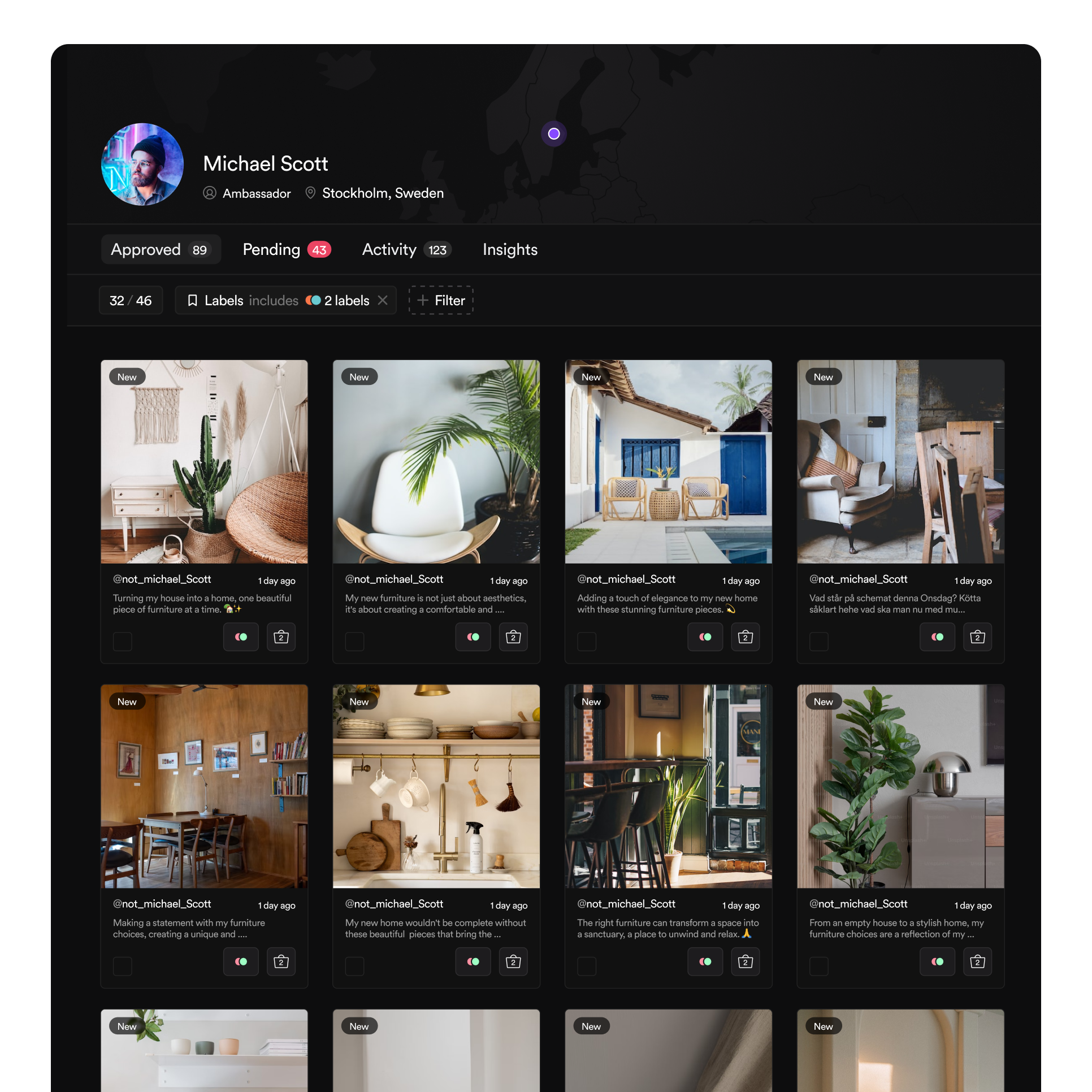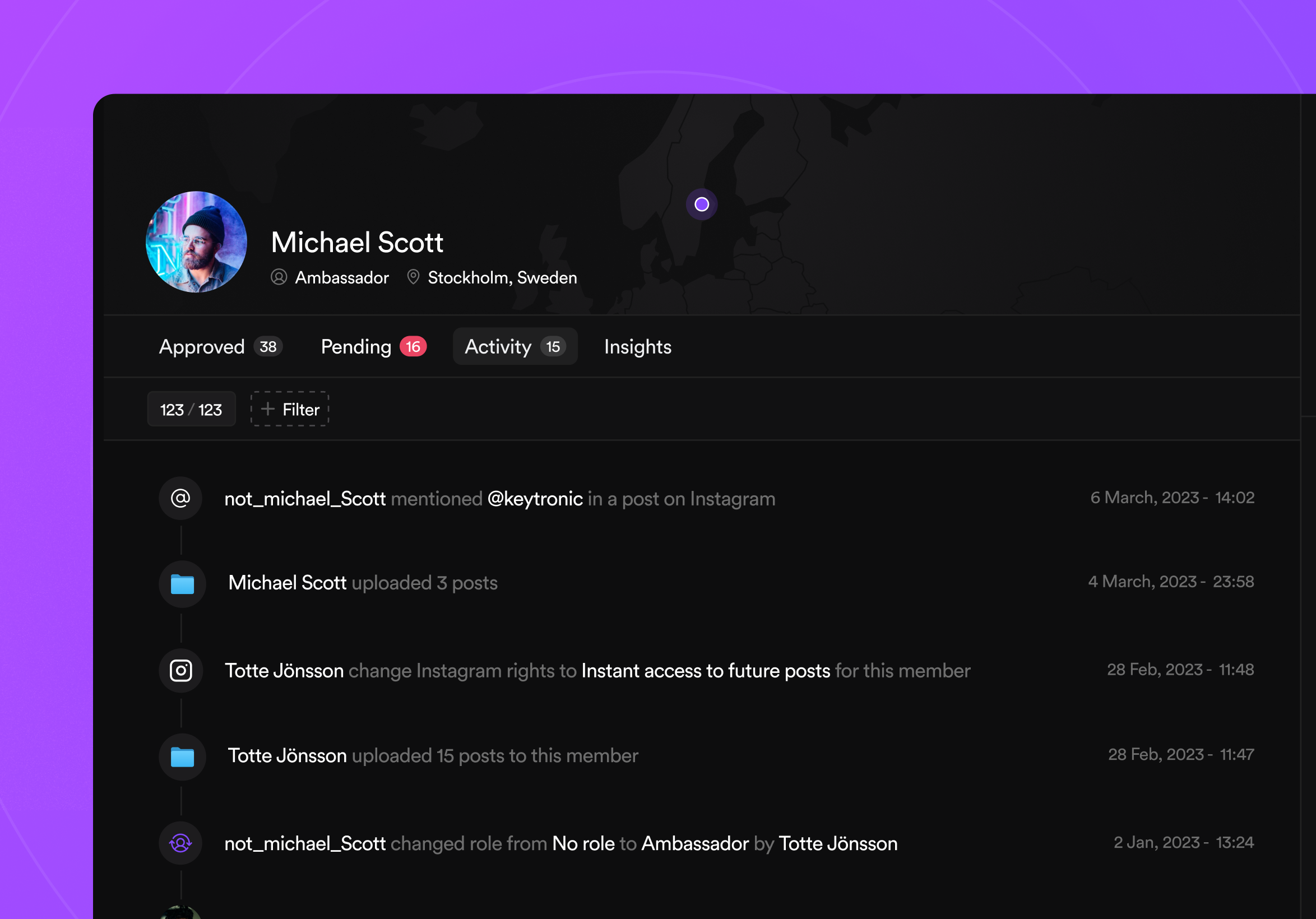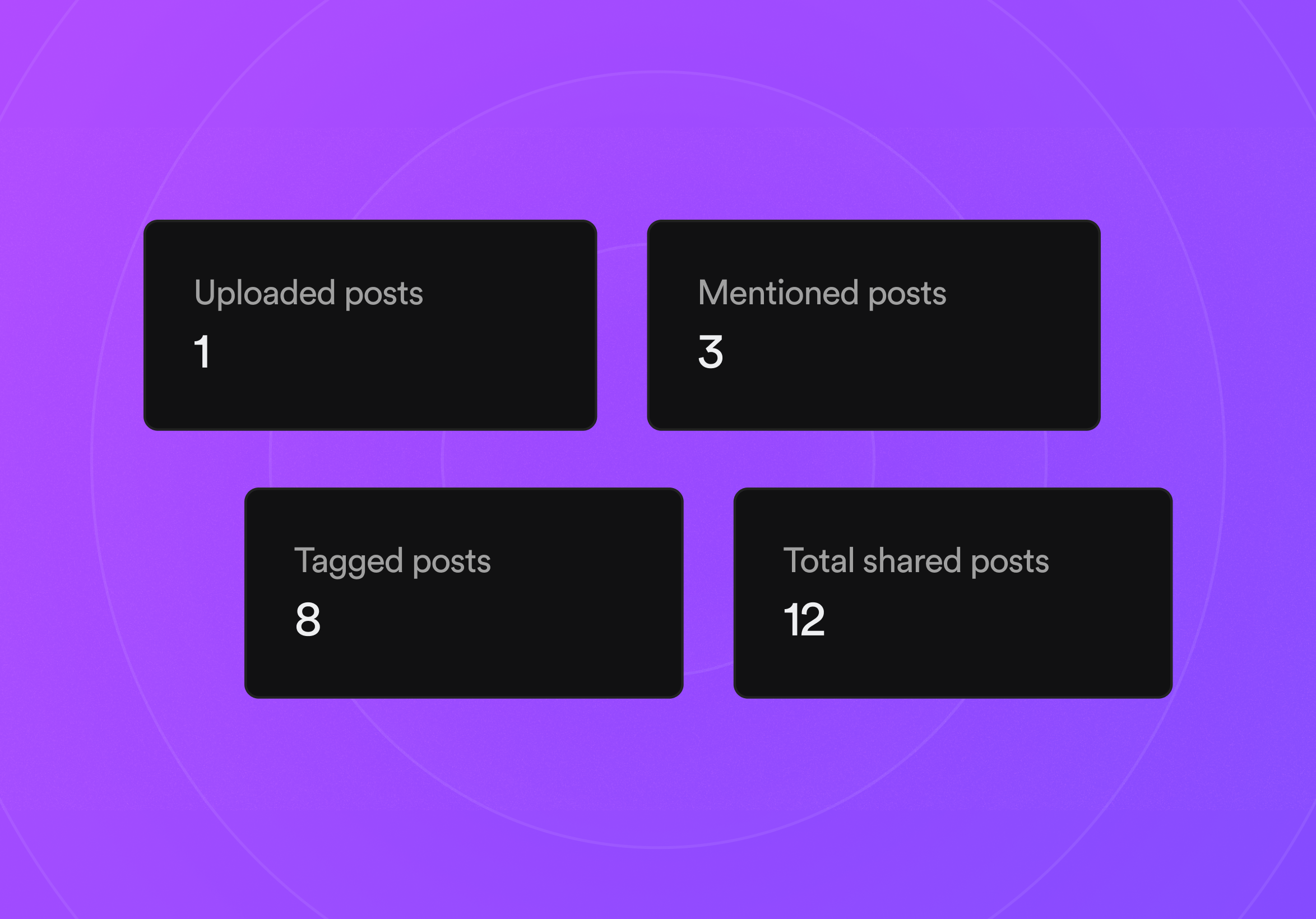Member Profile

# Find everything you need about each memberself.__wrap_n=self.__wrap_n||(self.CSS&&CSS.supports("text-wrap","balance")?1:2);self.__wrap_b=(t,n,e)=>{e=e||document.querySelector(`[data-br="\${t}"]`);let s=e.parentElement,r=O=>e.style.maxWidth=O+"px";e.style.maxWidth="";let o=s.clientWidth,u=s.clientHeight,a=o/2-.25,l=o+.5,d;if(o){for(r(a),a=Math.max(e.scrollWidth,a);a+1<l;)d=Math.round((a+l)/2),r(d),s.clientHeight===u?l=d:a=d;r(l*n+o*(1-n))}e.__wrap_o||(typeof ResizeObserver!="undefined"?(e.__wrap_o=new ResizeObserver(()=>{self.__wrap_b(0,+e.dataset.brr,e)})).observe(s):process.env.NODE_ENV==="development"&&console.warn("The browser you are using does not support the ResizeObserver API. Please consider add polyfill for this API to avoid potential layout shifts or upgrade your browser. Read more: https://github.com/shuding/react-wrap-balancer#browser-support-information"))};self.__wrap_n!=1&&self.__wrap_b("undefined",1)

## Get a convenient overview of each member’s content, activity, and creator infoContent

## Discover all content a member has sharedself.__wrap_n=self.__wrap_n||(self.CSS&&CSS.supports("text-wrap","balance")?1:2);self.__wrap_b=(t,n,e)=>{e=e||document.querySelector(`[data-br="\${t}"]`);let s=e.parentElement,r=O=>e.style.maxWidth=O+"px";e.style.maxWidth="";let o=s.clientWidth,u=s.clientHeight,a=o/2-.25,l=o+.5,d;if(o){for(r(a),a=Math.max(e.scrollWidth,a);a+1<l;)d=Math.round((a+l)/2),r(d),s.clientHeight===u?l=d:a=d;r(l*n+o*(1-n))}e.__wrap_o||(typeof ResizeObserver!="undefined"?(e.__wrap_o=new ResizeObserver(()=>{self.__wrap_b(0,+e.dataset.brr,e)})).observe(s):process.env.NODE_ENV==="development"&&console.warn("The browser you are using does not support the ResizeObserver API. Please consider add polyfill for this API to avoid potential layout shifts or upgrade your browser. Read more: https://github.com/shuding/react-wrap-balancer#browser-support-information"))};self.__wrap_n!=1&&self.__wrap_b("undefined",1)

Track your community members’ content from their profiles

Approved posts
Inbox
Explore members’ new posts that await your approval
Instagram
Track and request your members’ new Instagram posts
Activity

### Understand member activitiesself.__wrap_n=self.__wrap_n||(self.CSS&&CSS.supports("text-wrap","balance")?1:2);self.__wrap_b=(t,n,e)=>{e=e||document.querySelector(`[data-br="\${t}"]`);let s=e.parentElement,r=O=>e.style.maxWidth=O+"px";e.style.maxWidth="";let o=s.clientWidth,u=s.clientHeight,a=o/2-.25,l=o+.5,d;if(o){for(r(a),a=Math.max(e.scrollWidth,a);a+1<l;)d=Math.round((a+l)/2),r(d),s.clientHeight===u?l=d:a=d;r(l*n+o*(1-n))}e.__wrap_o||(typeof ResizeObserver!="undefined"?(e.__wrap_o=new ResizeObserver(()=>{self.__wrap_b(0,+e.dataset.brr,e)})).observe(s):process.env.NODE_ENV==="development"&&console.warn("The browser you are using does not support the ResizeObserver API. Please consider add polyfill for this API to avoid potential layout shifts or upgrade your browser. Read more: https://github.com/shuding/react-wrap-balancer#browser-support-information"))};self.__wrap_n!=1&&self.__wrap_b("undefined",1)Insights

### Check each member’s contributionsself.__wrap_n=self.__wrap_n||(self.CSS&&CSS.supports("text-wrap","balance")?1:2);self.__wrap_b=(t,n,e)=>{e=e||document.querySelector(`[data-br="\${t}"]`);let s=e.parentElement,r=O=>e.style.maxWidth=O+"px";e.style.maxWidth="";let o=s.clientWidth,u=s.clientHeight,a=o/2-.25,l=o+.5,d;if(o){for(r(a),a=Math.max(e.scrollWidth,a);a+1<l;)d=Math.round((a+l)/2),r(d),s.clientHeight===u?l=d:a=d;r(l*n+o*(1-n))}e.__wrap_o||(typeof ResizeObserver!="undefined"?(e.__wrap_o=new ResizeObserver(()=>{self.__wrap_b(0,+e.dataset.brr,e)})).observe(s):process.env.NODE_ENV==="development"&&console.warn("The browser you are using does not support the ResizeObserver API. Please consider add polyfill for this API to avoid potential layout shifts or upgrade your browser. Read more: https://github.com/shuding/react-wrap-balancer#browser-support-information"))};self.__wrap_n!=1&&self.__wrap_b("undefined",1)

See how many posts every member has uploaded and in how many they’ve tagged or mentioned you on InstagramMember info